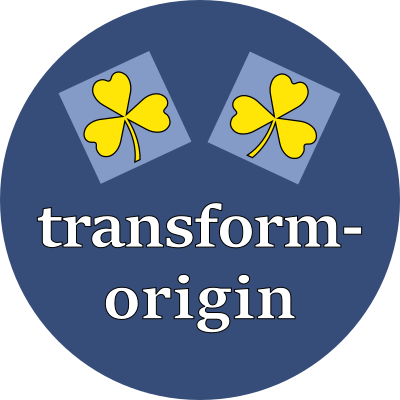# transform-originSpecify the point of reference for the transform operation.

## Property values

This property expects two values for 2D transformations, and three values for 3D transformations. The first two values may be a percentage, a distance, or one of the keywords that specify an edge. The third value must be a distance.

For 2D transformations, the first value is the offset from the element's left edge to the transformation's X origin. For convenience, these keywords may be used:

 left Use the left-edge as the X origin center Use the middle of the element as the X origin right Use the right-edge as the X origin

For 2D transformations, the second value is the offset from the element's top edge to the transformation's Y origin. For convenience, these keywords may be used:

 top Use the top-edge as the Y origin center Use the middle of the element as the Y origin bottom Use the bottom-edge as the Y origin

For 3D transformations, the third value is the offset from the plane of the screen to the user's point of reference, expressed as a distance (never a percentage).

## Examples

style > transform > transform-originTransform point of reference